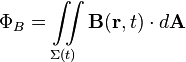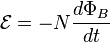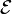# Faraday's law of induction facts for kids

Kids Encyclopedia Facts

Faraday's law of induction is a law of physics proposed by English physicist Michael Faraday in 1831. It is one of the basic laws of electromagnetism. The law explains the operation principles of generators, transformers and electrical motors.

Faraday's law of induction says that when a magnetic field changes, it causes a voltage. That phenomenon was also found by Joseph Henry in 1831. To describe the law, the magnetic flux and also a surface with a wire loop as border. This leads to the following surface integral:$\Phi_B = \iint\limits_{\Sigma(t)} \mathbf{B}(\mathbf{r}, t) \cdot d \mathbf{A}$

When the flux changes, it produces electromotive force. The flux changes when B changes or when the wire loop is moved or deformed, or when both happens. The electromotive force can then be calculated with the following equation:$\mathcal{E} = -N {{d\Phi_B} \over dt}$
•$\mathcal{E}$ is the electromotive force
• N is the number of loops the wire makes
• ΦB is the magnetic flux of one loop

## Images for kids

de:Elektromagnetische Induktion#Induktionsgesetz in IntegralformFaraday's law of induction Facts for Kids. Kiddle Encyclopedia.